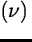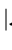Next: Sampling patterns Up: Fourier analysis: The sine Previous: Fourier transforms

The power spectrum and covariance statistics

Let us define power spectrum, covariance and autocovariance statistics P, Cov and ACF:=(12.7) Cov[x,y](l) = x(t)*y(-t) (12.8) ACF[x](l) = Cov[x,x](l) (12.9)

The power spectrum is special among the periodograms in that it is the square of a linear operator and reveals the important correspondence between frequency and time domain analyses:=(12.10)

by virtue of Eq. (12.5).

Let us consider which linear operators or matrices convert series of independent random variables into series of independent variables. For the discrete, evenly sampled observations the ACF is computed as the scalar product of vectors obtained by circularly permutating the data of the series. For a series of independent random variables, e.g. white noise, the vectors are orthogonal. It is known from linear algebra that only orthogonal matrices preserve orthogonality. So, only in the special case of evenly spaced discrete observations and frequencies (Sect. 12.3.1) are(and P[x]) independent for each frequency. In the next subsection we discuss the case of dependent and correlated values of P[x].Next: Sampling patterns Up: Fourier analysis: The sine Previous: Fourier transforms
Petra Nass
1999-06-15